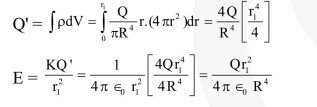Deepak Scored 45->99%ile with Bounce Back Crack Course. You can do it too!

# Prove the following

Question:

Let $\rho(\mathrm{r})=\frac{\mathrm{Q}}{\pi \mathrm{R}^{4}} \mathrm{r}$ be the charge density distribution for a solid sphere of radius $\mathrm{R}$ and total charge $\mathrm{Q}$. For a point 'p' inside the sphere at distance $r_{1}$ from the centre of the sphere, the magnitude of electric field is :-

1. $\frac{\mathrm{Qr}_{1}^{2}}{4 \pi \epsilon_{0} \mathrm{R}^{4}}$

2. $\frac{\mathrm{Qr}_{1}^{2}}{3 \pi \epsilon_{0} \mathrm{R}^{4}}$

3. 0

4. $\frac{Q}{4 \pi \epsilon_{0} r_{1}^{2}}$

Correct Option: 1

Solution: